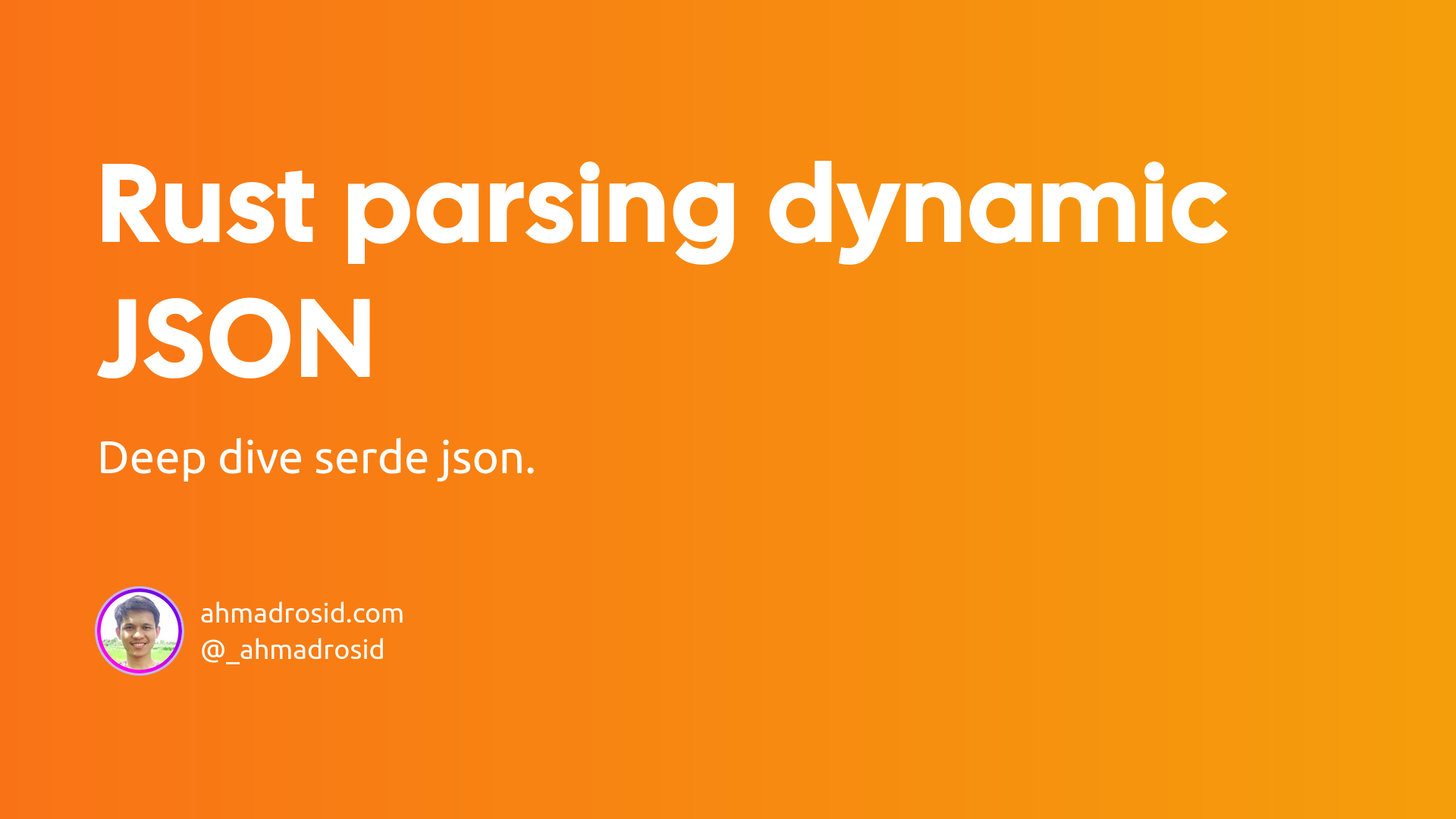# Rust parsing dynamic JSON

rustserdeserde_json

JSON is probably the most commonly used data structure. You can use it for configurations, databases, etc.

The best practice for json data structure is to have static key for each attribute. But sometime we need to deal with dynamic key.

## serde_json

In rust we are using serde_json library it has nice api to work with. So let's take a look how to parse json with serde_json.

Let's add serde dependency to Cargo.toml. We will use serde to serialize our data to struct.

``````serde = { version = "1.0", features = ["derive"] }
serde_json = "1.0"
``````

Now let's parse some json with static key properties.

``````use serde::{Deserialize, Serialize};
use serde_json::Result;

#[derive(Serialize, Deserialize)]
struct User {
age: usize,
email: String,
full_name: Vec<String>,
}

pub fn main() -> Result<()> {
let data = r#"
{
"age": 27,
"full_name": [
"Rosid"
]
}"#;

let u: User = serde_json::from_str(data)?;

println!("Hi {}", u.full_name);

Ok(())
}
``````

With static key property we can easily just declare the key and type to the struct. But if the key is dynamic we can not handle that.

## Dynamic Json

Luckily serde_json have this enum to work with json data structure.

``````enum Value {
Null,
Bool(bool),
Number(Number),
String(String),
Array(Vec<Value>),
Object(Map<String, Value>),
}
``````

With this enum we can check every key and do some serialization on that. Now, suppose we have this json.

``````{
"plugins": {
"width": [["w",["width"]]],
"height": [["h",["height"]]],
"z_index": [["z",["z-index"]]],
}
}
``````

It seems simple right? We can easily declare the struct like this.

``````#[derive(Serialize, Deserialize)]
struct Data {
plugins: Plugin,
}

#[derive(Serialize, Deserialize)]
struct Plugin {
width: Vec<Value>,
height: Vec<Value>,
z_index: Vec<Value>,
}
``````

But what if the key inside `plugins` is dynamic for example what if the `z_index` become `z-index` and the keys is more that those three in the example.

So to handle that case we need to turn this data to `Map<String, Value>`. Now we can write our struct to become like this.

``````#[derive(Serialize, Deserialize)]
struct Data {
plugins: Map<String, Value>,
}
``````

Now we can access the data like this.

``````let data: Data = serde_json::from_str(json)?;
let z_index: Value = data.get("z-index").unwrap();
``````

To turn the `Value` to array we can do it like this.

``````let z_index_arr: Vec<&Value> = z_index.as_array().unwrap();
``````

We also can improve by transforming the value inside z_index which is an array to key value. As you can see the value of `z_index` is array inside array.

``````[
[
"z",
[
"z-index"
]
]
]
``````

Let's turn this value to:

``````{
"z": ["z-index"]
}
``````

To do that we will work with Map<String, Value> directly.

``````let z_index: Vec<&Value> = data.get("z-index").unwrap().as_array().unwrap();
let mut data = Map::new();

for item in z_index {
if item.get(0)?.is_string() {
let key = item.get(0)?.as_str()?.to_string();
let variants = item.get(1)?.clone();
}
}
``````

Pro tip! If you don't want to use `unwrap()` every time you convert enum `Value` just use ? and wrap your function with Option<()>

Now we can access the value of `z-index` like this.

``````let arr: Vec<&Value> = data.get("z")?;
``````

I think that's all, more documentation for serde here.

Have any question? Connect with me in twitter